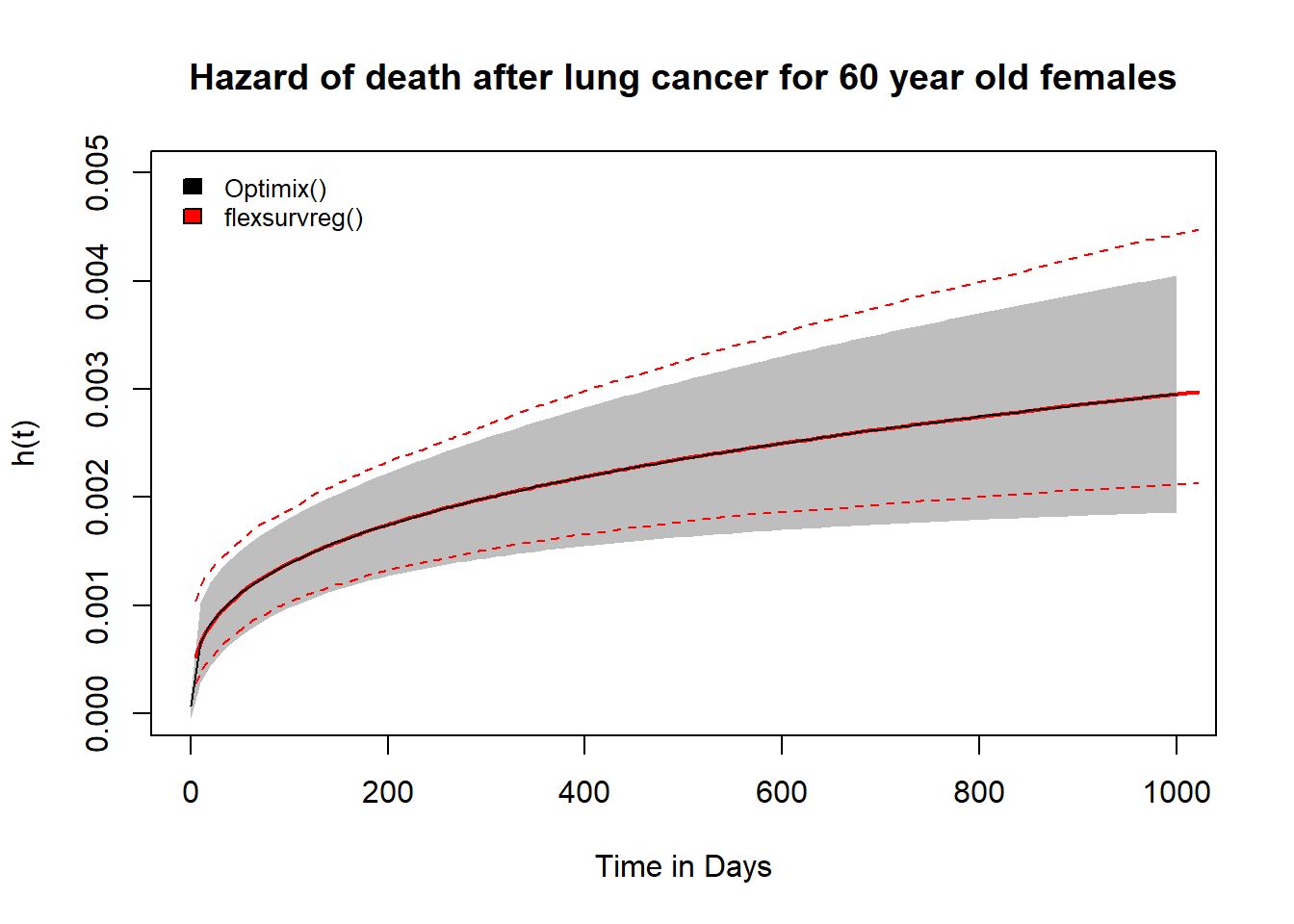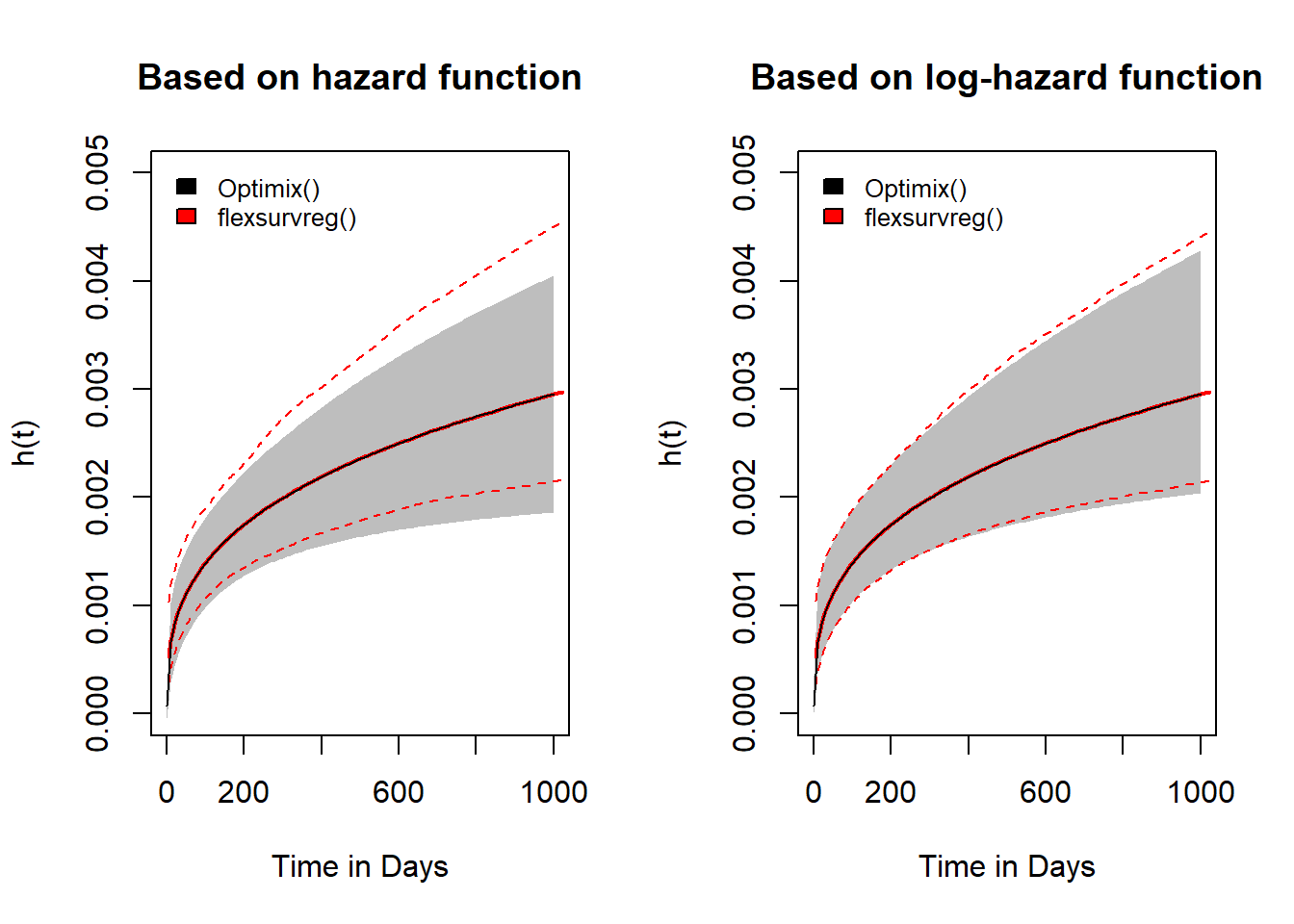# Comparing the confidence intervals of a Weibull model estimated with flexsurvreg() and optimx()

This blog post is a follow up on my previous post on optimising a Weibull regression model using optimx(). This time I’ll try to find a solution for the discrepancy between the confidence interval estimates of the Weibull hazard function estimated with optimx() and flexsurvreg().

This post begins where my previous one ended. Hence, I suggest you to read my previous post before reading this one. Also, I will use the R script of my previous post as starting point for this post. You can find the whole plain R script used in this post in .txt format here.This is the figure where my last post ended. I compared the hazard function $$h(t)$$ of the Weibull model estimated manually using optimx() with the hazard function of an identical model estimated with flexsurvreg(). Interestingly, the hazard functions were identical, but there were considerable differences in the estimates of the confidence intervals across follow-up time, as you can see in the figure above. Unfortunately, I couldn’t come up with an explanation for this discrepancy. However, I was lucky and got help from Andrea Discacciati, a colleague at Karolinska Institutet. He pointed out that the flexsurvreg() function from the {flexsurvreg} package uses the log-hazard function ($$\ln h(t)$$) for estimating confidence intervals whereas I used the hazard function ($$h(t)$$) in my post instead. Working on the log-scale allows to transform the multiplicative components of the hazard function into additive components, since $$\ln xy = \ln x + \ln y$$. This is usually done to increase the precision of the computation process, since multiplication usually comes with a higher loss in precision compared to summation. Hence, using the hazard function instead of the log-hazard function might lead to the difference in the estimates for the confidence intervals of the hazard functions, that we can see in the figure above.

So let’s try to use the log-hazard instead of the hazard for the computation of our survival function together with its confidence intervals. First the hazard function is, as explained in my previous post, defined by

$h(t) = \gamma \lambda t ^{\gamma - 1}.$

Let’s then compute the hazard function and the confidence intervals using the hazard function on the identity scale.

# 12. Compare h(t) with CIs using identity and log-scale ----------------------

# Get coefficents for Newuoa optimisation
newuoa_coef <- coef(opt)["newuoa", ]

# 12.1 Estimate h(t) with CIs using hazard function on identity scale =========

# Compute CIs for a 60 year old female across time
haz_optim_female <- lapply(as.list(seq(0.01, 1000.01, 10)), function(t){

g <- paste("exp(gamma) * exp(alpha + beta_female + 60 * beta_age) *", t,
"^ (exp(gamma) - 1)")

fit <- deltaMethod(newuoa_coef, g, solve(hessian_m))

data.frame(time     = t,
estimate = fit[, "Estimate"],
ci_low   = fit[, "2.5 %"],
ci_up    = fit[, "97.5 %"])

}) %>%
bind_rows()

In the next step we need to compute the hazard function and its confidence interval on the log-scale $$\ln h(t)$$. The log-hazard function can be written as

$\ln h(t) = \ln \gamma + \ln \lambda + \ln t (\exp(\gamma) - 1).$

Let’s use this formula to calculate the confidence interval of the hazard function using the same function as above.

# 12.2 Estimate h(t) with CIs using hazard function on log scale ==============

# Compute CIs for a 60 year old female across time
haz_optim_female_log <- lapply(as.list(seq(0.01, 1000.01, 10)), function(t){

g <- paste("(gamma) + (alpha + beta_female + 60 * beta_age) +", log(t),
"* (exp(gamma) - 1)")

fit <- deltaMethod(newuoa_coef, g, solve(hessian_m))

data.frame(time     = t,
estimate = exp(fit[, "Estimate"]),
ci_low   = exp(fit[, "2.5 %"]),
ci_up    = exp(fit[, "97.5 %"]))

}) %>%
bind_rows()

We can now compare the confidence intervals obtained by these two different computations by plotting their hazard functions and confidence intervals.

# 12.3 Create plot comparing both estimations =================================

par(mfcol = c(1, 2))

# 10.2 Plot hazard curve with CIs =============================================

# create list of both data frames

list_haz_optim_female <- list(haz_optim_female,
haz_optim_female_log)

invisible(
mapply(df = list(haz_optim_female, haz_optim_female_log),
titles = c("Based on hazard function",
"Based on log-hazard function"),
FUN = function(df, titles){

plot(df$time, df$estimate,
ylim = c(0, 0.005),
type = "n",
xlab = "Time in Days",
ylab = "h(t)",
main = titles)
polygon(c(df$time, rev(df$time)),
c(df$ci_low, rev(df$ci_up)),
border = NA,
col = "grey")
plot(weibull_model, type = "hazard",
newdata = data.frame(age = 60,
female = 1),
lines(df$time, df$estimate)
legend("topleft",
inset = 0.01,
cex = 0.8,
fill = c("black", "red"),
legend = c("Optimix()", "flexsurvreg()"),
box.lty = 0)
})
)In this figure we can see the hazard function and its confidence interval (gray area) for both computation approaches. The estimates yielded by working on the identity scale are shwon on the left and the estimates yieled by working on the log-scale are shown on the right. At the same time we see the estimates yielded by estimating the same model with flexsurvreg() (red lines). As we can see, working on the log-scale yielded estimates very much closer to the estimates obtained with flexsurvreg() compared to the model using the identity scale.

Hence, it seems as this difference between working on the identity and log-scale for computing the confidence intervals may explain a big part of the discrepancy between the confidence intervals obtained with flexsurvreg() and optimx(), which we can see in the first figure above. The remaining differences are likely due to the fact, that the flexsurvreg() function uses the bootstrap method instead of the delta method to estimate confidence intervals for the hazard function.

In this post we compared the confidence intervals of a hazard function obtained by applying the delta method to the hazard function on the identity scale ($$h(t)$$) and on the log-scale ($$\ln h(t)$$). As Andrea Discacciati pointed out both approaches assymptotically yield the same results. However, working on the log-hazard scale is favorable for finite samples.